# Problems of the Week

Contribute a problem

# 2018-01-15 Intermediate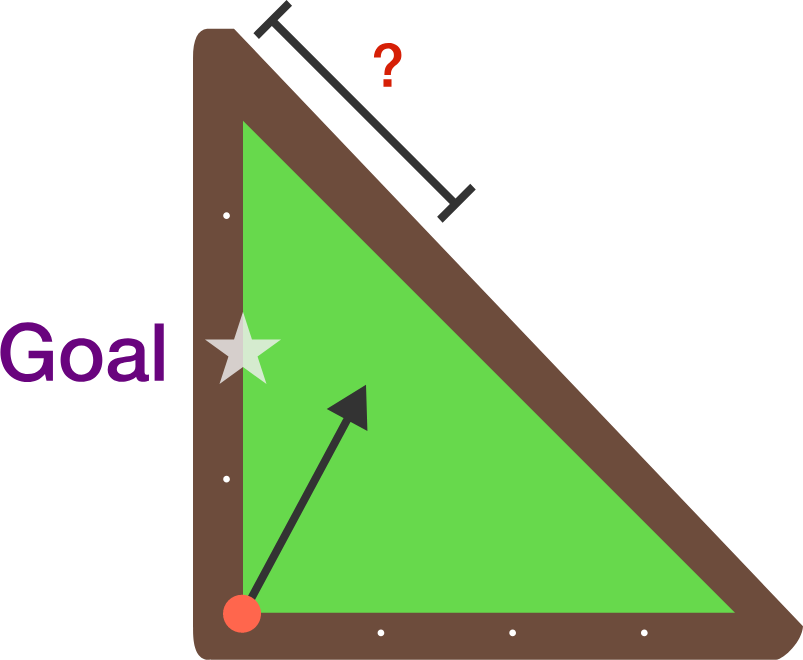If you have a pool table as shown shaped like an isosceles right triangle, with a ball that starts at the right angle, where should you aim at on the hypotenuse so after one bounce the ball hits the midpoint of the left-hand side of the table?

This problem is part of the Brilliant.org Open Problems Group. The end goal for each open problem is to find a solution, and maybe publish it if it's a nice enough result! You can read about the unsolved billiards problem that this one is related to here.

Assume geometric purity: the "ball" has no mass, the "ball" and "hole" are considered single points, and the ball travels straight.

A car and a truck are traveling in a row at the same speed of 90 km/h.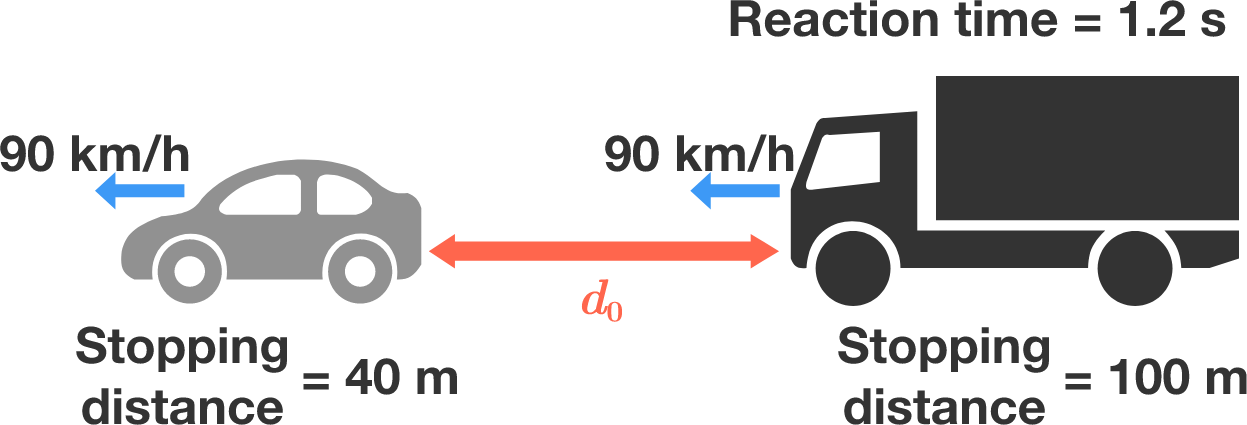Suddenly, the car brakes to a complete stop, and then the truck comes to a complete stop to avoid hitting the car. How far apart (in meters) should the two vehicles be so that the truck avoids crashing into the car?

Details and Assumptions:

• The car takes 40 m to brake to a complete stop, and the truck takes 100 m to brake to a complete stop.
• The truck driver takes 1.2 seconds to react to the car braking.
• The front of the truck will just barely touch the back of the car after the truck finishes stopping.

Bonus problem: How big should the safety distance be if the truck drives in front of the car? Assume that the car driver has the same reaction time of 1.2 s.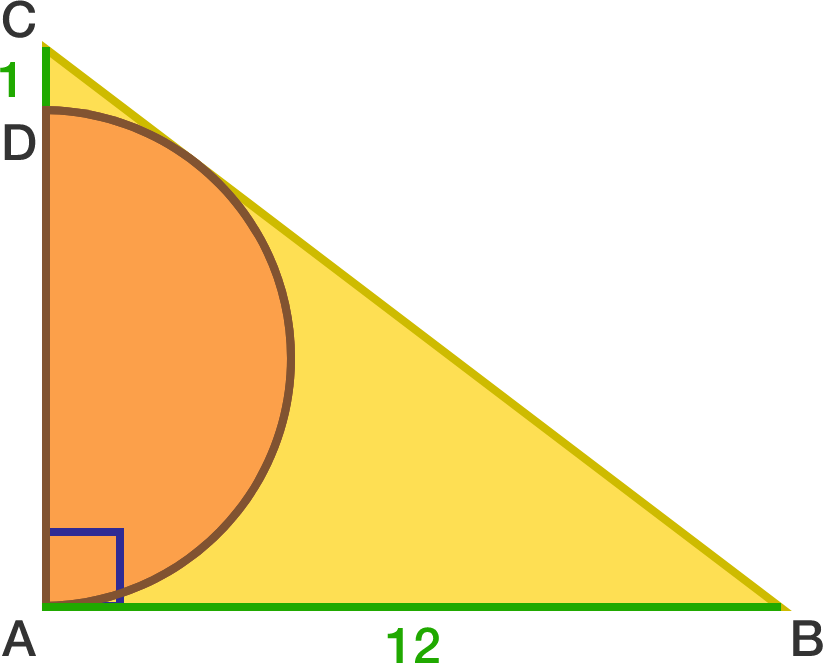Given that $AB=12, CD=1, \angle BAC = 90^\circ,$ and the semicircle is tangent to $BC,$ find the radius of the semicircle.

An ant begins from $(0,0)$ in the $xy$-coordinate plane. It then travels to each point with non-negative integer coordinates following the pattern shown below.

If the ant stops moving after $2018$ units, find its final position $(x,y)$ and enter your answer as $x+y.$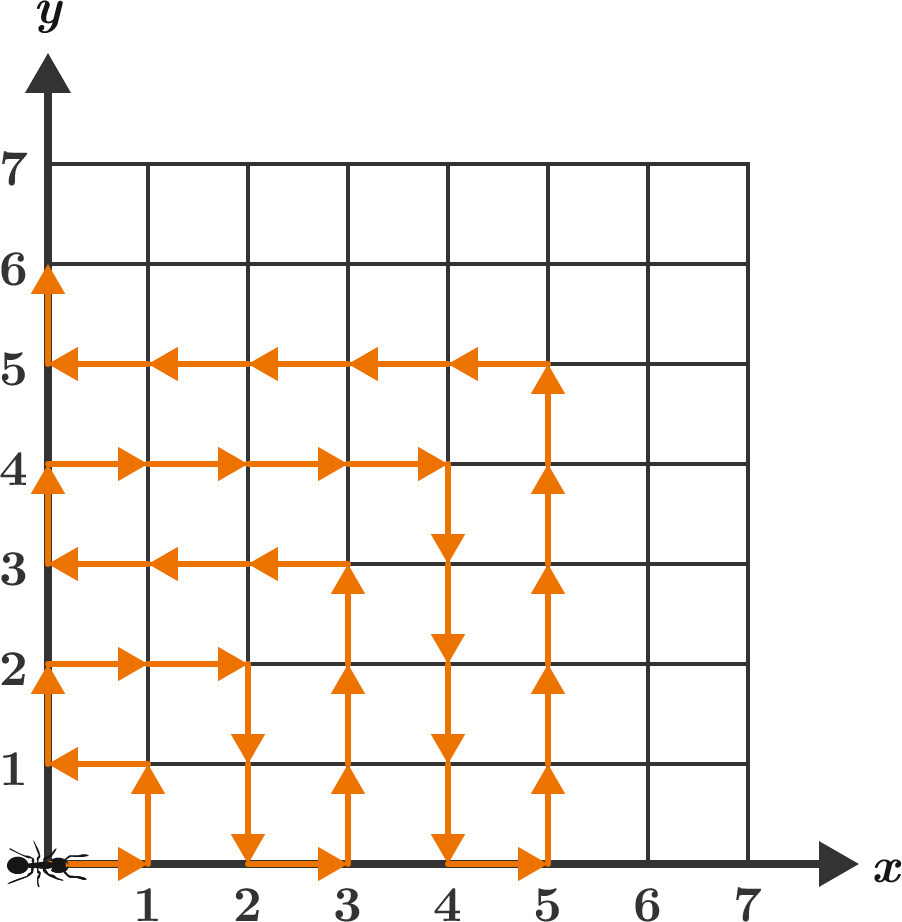There is a light in another room that can be in one of three states: $\color{#3D99F6}\text{blue},$ $\color{#D61F06}\text{red},$ or $\text{off}.$

In your room, you have two buttons numbered 1 and 2 that control the state of the light. The state of light changes according to the diagram below.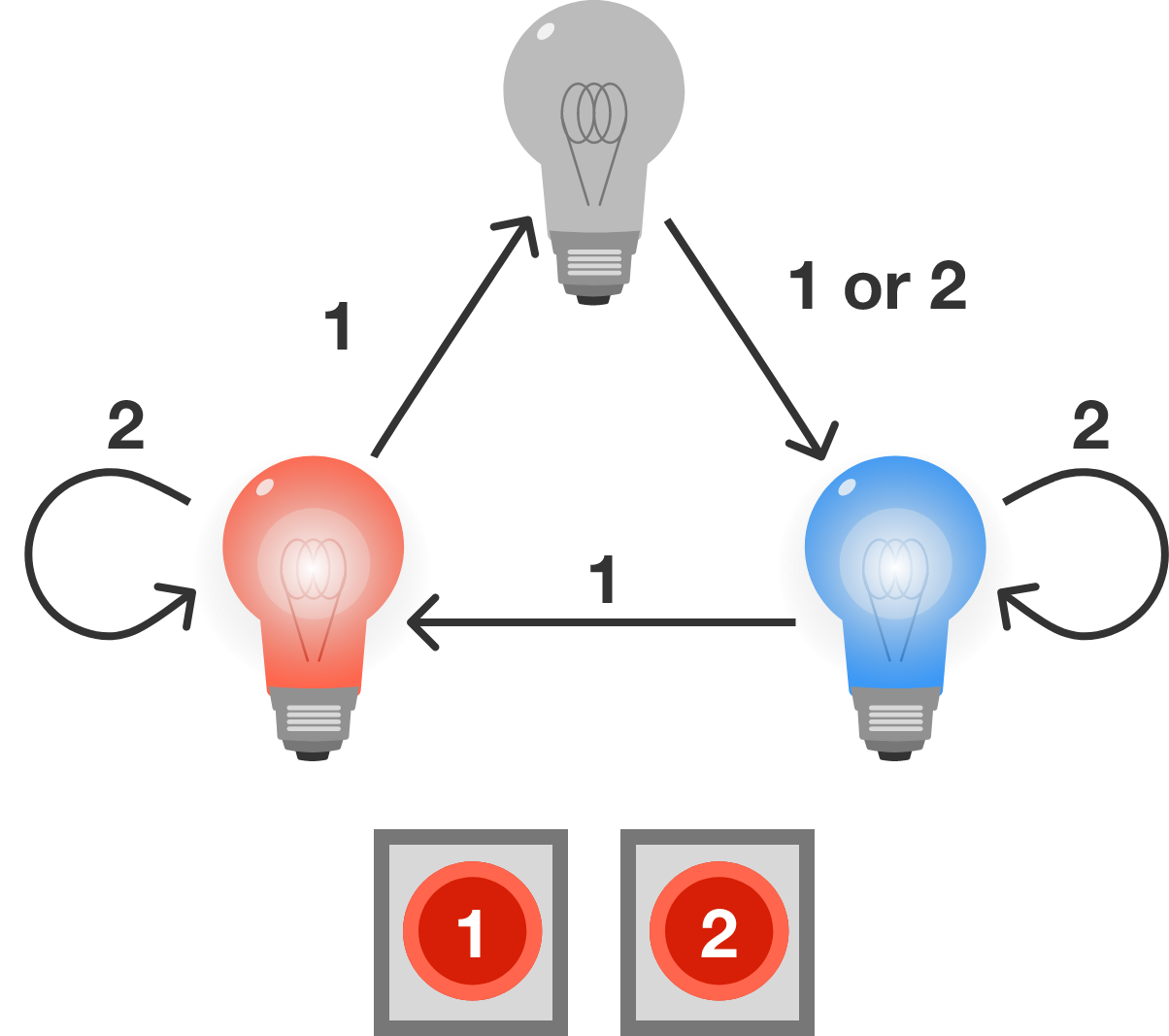• If you press button 1, then the light changes to the next state clockwise around the diagram.
• If you press button 2 while the light is $\color{#3D99F6}\text{blue}$ or $\color{#D61F06}\text{red},$ the state of the light will not change.
• If you press button 2 while the light is $\text{off},$ the light will change to $\color{#3D99F6}\text{blue}.$

You want to make sure that the light is $\text{off},$ but since the light is in another room, you do not know what state the light is currently in.

What is the shortest sequence of button presses that will guarantee that the light will be $\text{off}?$

Note: Enter your answer as a sequence of digits in order. For example, if you believe the sequence is {1, 2, 1, 2, 1}, then enter $12121.$

×